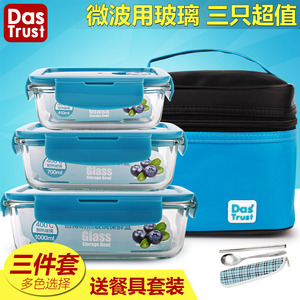### 创得玻璃饭盒微波炉专用保鲜盒饭盒套装便当盒带盖长方圆形保鲜碗(包邮)• 优惠价：29.90-49.90元
• 原价:128.00元

## 相关参数

• 包装体积1
• 品牌创得（家居）
• 型号DERGLB单多套装
• 形状多规格套装
• 颜色分类绿圆400+650+1000,紫圆400+650+1000,蓝圆400+650+1000,白色圆400+650+1000,绿正350+500+750,紫正350+500+750,湖蓝长410+700+1000,北欧绿长410+700+1000,绿长410+700+1000,紫长410+700+1000,蓝长410+700+1000,蓝650+650+包,紫长700+分隔800+包,湖蓝700+700+包,湖蓝500+500+包,湖蓝650+700+包,北欧绿分隔500+分隔800+包,紫500+分隔正1050+包,绿长410+700+1000+包,紫长410+700+1000+包,蓝长410+700+1000+包,湖蓝长410+700+1000+包,绿700+分隔800+长1000+包,紫700+分隔800+长1000+包
• 容量1L及以上
• 材质耐热玻璃
• 包装数量3个
• 产地中国
• 尺寸多规格套装
• 主图来源自主实拍图

## 更多相关优惠券## [看看用户的体验都是啥样的]

mall.yzhbw.net-点击查看商品详情